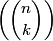# Four uncommon but handy math notations

Here are some of my favorite notations that are not commonly used.

The first is Richard Stanley’s notation for counting the number of ways to select k objects from a set of n objects with replacement. This is similar to the problem solved by binomial coefficients, but not the same since binomial coefficients count the number of possible selections without replacement. Stanley’s symbol isI like this symbol for two reasons. First, it’s good to have a notation, any notation, for a concept that comes up fairly often. Second, it’s appropriate for this symbol to resemble the binary coefficient symbol. See selecting with replacement for more on Stanley’s symbol, how to think about it and how to compute it.

Next is Kenneth Iverson’s notation for indicator functions. Iverson’s idea was to put a Boolean condition in square brackets to indicate the function that is 1 when that condition is true and 0 otherwise. For example, [x > y] is the function f(x, y) such that f equals 1 when x is greater than y and equals 0 for all other arguments. This notation saves ink and makes it easier to concentrate on the substance of an expression. For more on Iverson’s notation, see Concrete Mathematics.

Another notation from Concrete Mathematics is the use of a perpendicular symbol to note that two integers are relatively prime. For example, mn would indicate that that m and n are relatively prime. The more common way to denote this would be to say gcd(m, n) = 1. The perpendicular symbol is nice because perpendicular lines have no component of direction in common, just as relative prime numbers have no prime factors in common.

Finally, multi-index notation is a handy way to make multivariable theorems easier to remember. For example, with this notation, Taylor series in several variables look similar to Taylor series in one variable.

## 3 thoughts on “Four uncommon but handy math notations”

1. Jack Kennedy

Like that multi-index notation.

Another useful notation from Concrete Mathematics:
the falling factorial.
I’d show an example, but I’m not sure if I can type LaTeX or fancy HTML here.

2. Jack, unfortunately the blogging software doesn’t support LaTeX notation, but here’s an example of the notation you’re talking about. These notes on hypergeometric functions being with defining rising and falling factorial powers.

3. I would have liked the multi-index notation, too, had you shown us…

If you allow your commentors to embed images, we can make latex work.

There is an alternative, actually. If you enable ASCIIMathML… you would need to tweak your HTML code to embed a particular javascript code. And that is it! Anyone who types Latex inside of dollar signs will get a regular unicode based pretty math expression.